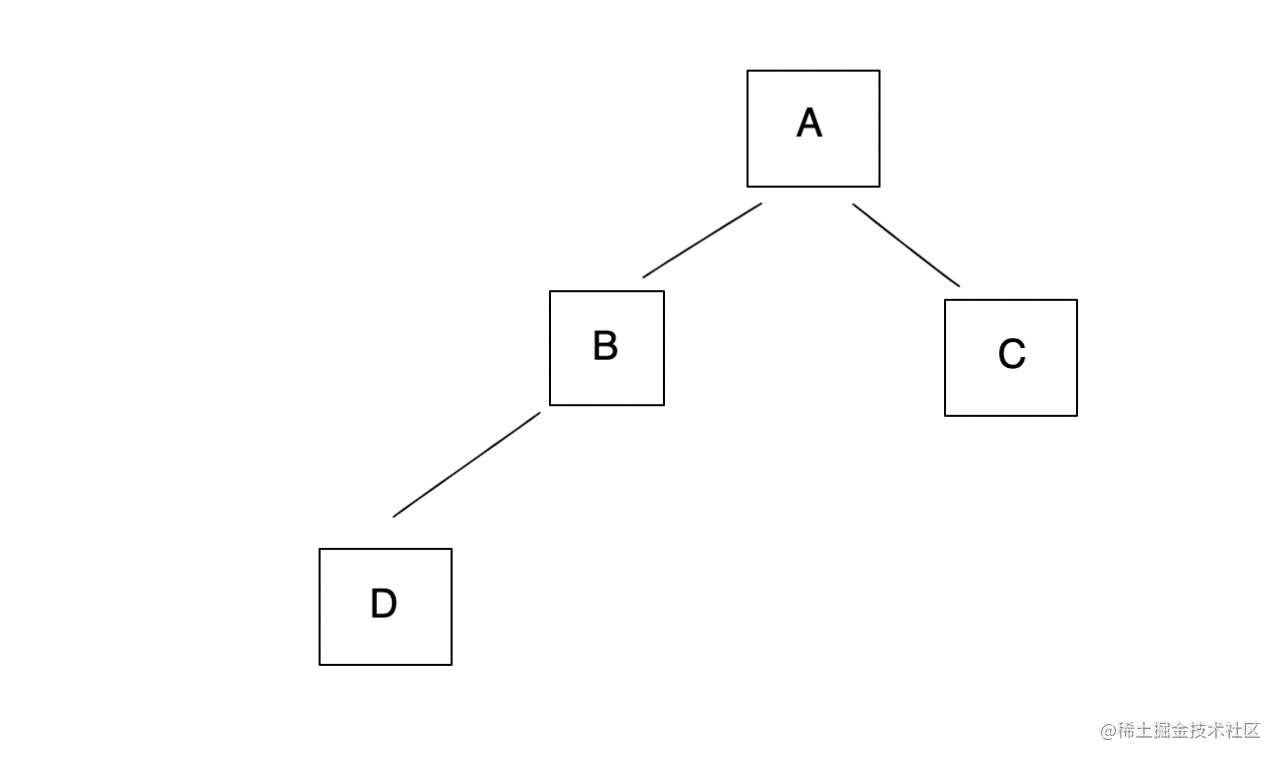# 算法：二叉树的层序遍历

「这是我参与11月更文挑战的第14天，活动详情查看：2021最后一次更文挑战

Hope is a good thing, maybe the best of things. And no good thing ever dies.

## 什么是层序遍历• 前序遍历：A → B → D → C
• 中序遍历：B → D → A → C
• 后续遍历：D → B → C → A
• 层序遍历：A → B → C → D

## 题目

``````示例：

3
/ \
9  20
/  \
15   7

[
,
[9,20],
[15,7]
]

## 解题

``````var levelOrder = function (root) {
if (root == null) {
return [];
}

let result = [];
let queue = [root];

while (queue.length) {
let levelSize = queue.length;

// 当前层级的结果
let curLevelResult = [];

// 遍历当前层级的结果
for (let i = 0; i < levelSize; i++) {
const node = queue.shift();
curLevelResult.push(node.val);
if (node.left) queue.push(node.left);
if (node.right) queue.push(node.right);
}

result.push(curLevelResult);
}

return result;
};